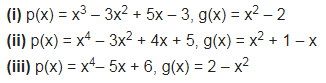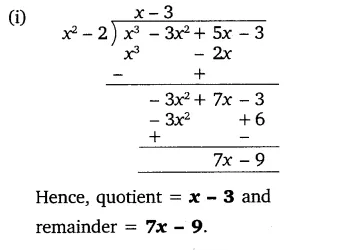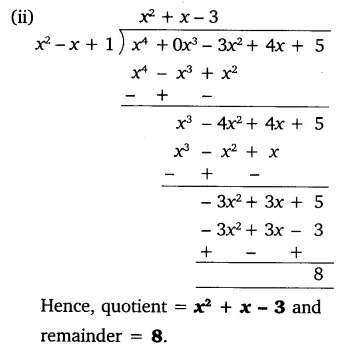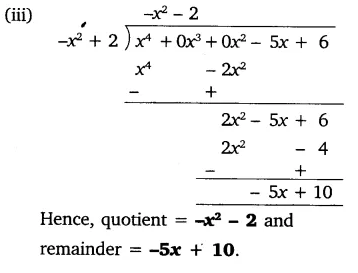# Divide the polynomial p(x) by the polynomial g(x) and find the quotient and remainder in

Divide the polynomial p(x) by the polynomial g(x) and find the quotient and remainder in each of the following: